# 12.4 Rotation of axes  (Page 2/8)

 Page 2 / 8

## Identifying a conic from its general form

Identify the graph of each of the following nondegenerate conic sections.

1. $4{x}^{2}-9{y}^{2}+36x+36y-125=0$
2. $9{y}^{2}+16x+36y-10=0$
3. $3{x}^{2}+3{y}^{2}-2x-6y-4=0$
4. $-25{x}^{2}-4{y}^{2}+100x+16y+20=0$
1. Rewriting the general form, we have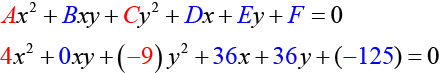$A=4\text{\hspace{0.17em}}$ and $\text{\hspace{0.17em}}C=-9,$ so we observe that $\text{\hspace{0.17em}}A\text{\hspace{0.17em}}$ and $\text{\hspace{0.17em}}C\text{\hspace{0.17em}}$ have opposite signs. The graph of this equation is a hyperbola.

2. Rewriting the general form, we have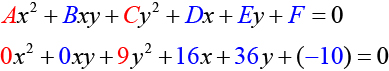$A=0\text{\hspace{0.17em}}$ and $\text{\hspace{0.17em}}C=9.\text{\hspace{0.17em}}$ We can determine that the equation is a parabola, since $\text{\hspace{0.17em}}A\text{\hspace{0.17em}}$ is zero.

3. Rewriting the general form, we have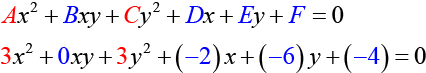$A=3\text{\hspace{0.17em}}$ and $\text{\hspace{0.17em}}C=3.\text{\hspace{0.17em}}$ Because $\text{\hspace{0.17em}}A=C,$ the graph of this equation is a circle.

4. Rewriting the general form, we have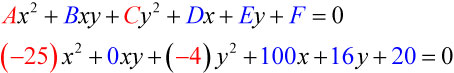$A=-25\text{\hspace{0.17em}}$ and $\text{\hspace{0.17em}}C=-4.\text{\hspace{0.17em}}$ Because $\text{\hspace{0.17em}}AC>0\text{\hspace{0.17em}}$ and $\text{\hspace{0.17em}}A\ne C,$ the graph of this equation is an ellipse.

Identify the graph of each of the following nondegenerate conic sections.

1. $16{y}^{2}-{x}^{2}+x-4y-9=0$
2. $16{x}^{2}+4{y}^{2}+16x+49y-81=0$
1. hyperbola
2. ellipse

## Finding a new representation of the given equation after rotating through a given angle

Until now, we have looked at equations of conic sections without an $\text{\hspace{0.17em}}xy\text{\hspace{0.17em}}$ term, which aligns the graphs with the x - and y -axes. When we add an $\text{\hspace{0.17em}}xy\text{\hspace{0.17em}}$ term, we are rotating the conic about the origin. If the x - and y -axes are rotated through an angle, say $\text{\hspace{0.17em}}\theta ,$ then every point on the plane may be thought of as having two representations: $\text{\hspace{0.17em}}\left(x,y\right)\text{\hspace{0.17em}}$ on the Cartesian plane with the original x -axis and y -axis, and $\text{\hspace{0.17em}}\left({x}^{\prime },{y}^{\prime }\right)\text{\hspace{0.17em}}$ on the new plane defined by the new, rotated axes, called the x' -axis and y' -axis. See [link] .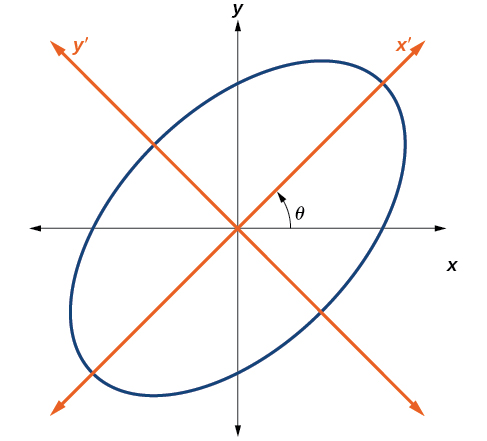The graph of the rotated ellipse   x 2 + y 2 – x y – 15 = 0

We will find the relationships between $\text{\hspace{0.17em}}x\text{\hspace{0.17em}}$ and $\text{\hspace{0.17em}}y\text{\hspace{0.17em}}$ on the Cartesian plane with $\text{\hspace{0.17em}}{x}^{\prime }\text{\hspace{0.17em}}$ and $\text{\hspace{0.17em}}{y}^{\prime }\text{\hspace{0.17em}}$ on the new rotated plane. See [link] .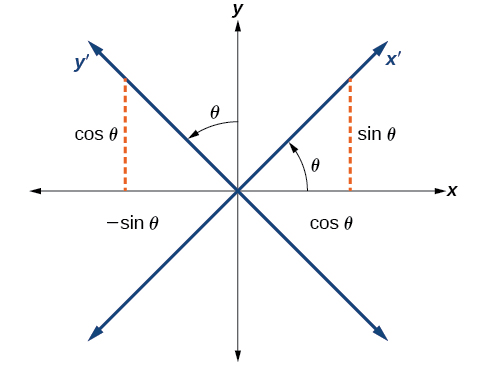The Cartesian plane with x - and y -axes and the resulting x ′− and y ′−axes formed by a rotation by an angle   θ .

The original coordinate x - and y -axes have unit vectors $\text{\hspace{0.17em}}i\text{\hspace{0.17em}}$ and $\text{\hspace{0.17em}}j\text{\hspace{0.17em}}.$ The rotated coordinate axes have unit vectors $\text{\hspace{0.17em}}{i}^{\prime }\text{\hspace{0.17em}}$ and $\text{\hspace{0.17em}}{j}^{\prime }.$ The angle $\text{\hspace{0.17em}}\theta \text{\hspace{0.17em}}$ is known as the angle of rotation    . See [link] . We may write the new unit vectors in terms of the original ones.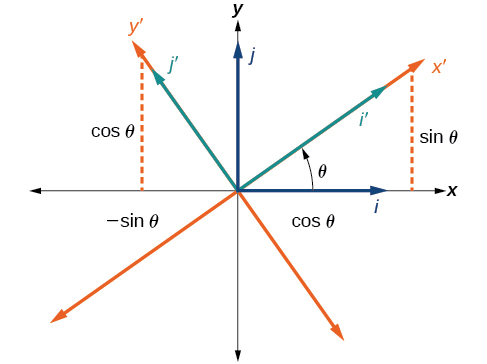Relationship between the old and new coordinate planes.

Consider a vector $\text{\hspace{0.17em}}u\text{\hspace{0.17em}}$ in the new coordinate plane. It may be represented in terms of its coordinate axes.

Because $\text{\hspace{0.17em}}u={x}^{\prime }{i}^{\prime }+{y}^{\prime }{j}^{\prime },$ we have representations of $\text{\hspace{0.17em}}x\text{\hspace{0.17em}}$ and $\text{\hspace{0.17em}}y\text{\hspace{0.17em}}$ in terms of the new coordinate system.

## Equations of rotation

If a point $\text{\hspace{0.17em}}\left(x,y\right)\text{\hspace{0.17em}}$ on the Cartesian plane is represented on a new coordinate plane where the axes of rotation are formed by rotating an angle $\text{\hspace{0.17em}}\theta \text{\hspace{0.17em}}$ from the positive x -axis, then the coordinates of the point with respect to the new axes are $\text{\hspace{0.17em}}\left({x}^{\prime },{y}^{\prime }\right).\text{\hspace{0.17em}}$ We can use the following equations of rotation to define the relationship between $\text{\hspace{0.17em}}\left(x,y\right)\text{\hspace{0.17em}}$ and $\text{\hspace{0.17em}}\left({x}^{\prime },{y}^{\prime }\right):$

and

Given the equation of a conic, find a new representation after rotating through an angle.

1. Find $\text{\hspace{0.17em}}x\text{\hspace{0.17em}}$ and $\text{\hspace{0.17em}}y\text{\hspace{0.17em}}$ where and
2. Substitute the expression for $\text{\hspace{0.17em}}x\text{\hspace{0.17em}}$ and $\text{\hspace{0.17em}}y\text{\hspace{0.17em}}$ into in the given equation, then simplify.
3. Write the equations with $\text{\hspace{0.17em}}{x}^{\prime }\text{\hspace{0.17em}}$ and $\text{\hspace{0.17em}}{y}^{\prime }\text{\hspace{0.17em}}$ in standard form.

#### Questions & Answers

write down the polynomial function with root 1/3,2,-3 with solution
if A and B are subspaces of V prove that (A+B)/B=A/(A-B)
write down the value of each of the following in surd form a)cos(-65°) b)sin(-180°)c)tan(225°)d)tan(135°)
Prove that (sinA/1-cosA - 1-cosA/sinA) (cosA/1-sinA - 1-sinA/cosA) = 4
what is the answer to dividing negative index
In a triangle ABC prove that. (b+c)cosA+(c+a)cosB+(a+b)cisC=a+b+c.
give me the waec 2019 questions
the polar co-ordinate of the point (-1, -1)
prove the identites sin x ( 1+ tan x )+ cos x ( 1+ cot x )= sec x + cosec x
tanh`(x-iy) =A+iB, find A and B
B=Ai-itan(hx-hiy)
Rukmini
what is the addition of 101011 with 101010
If those numbers are binary, it's 1010101. If they are base 10, it's 202021.
Jack
extra power 4 minus 5 x cube + 7 x square minus 5 x + 1 equal to zero
the gradient function of a curve is 2x+4 and the curve passes through point (1,4) find the equation of the curve
1+cos²A/cos²A=2cosec²A-1
test for convergence the series 1+x/2+2!/9x3ByBy